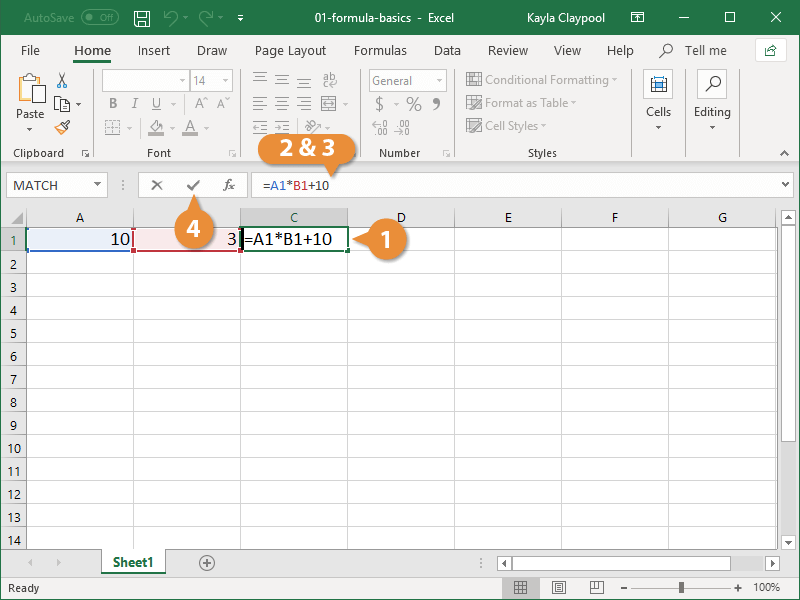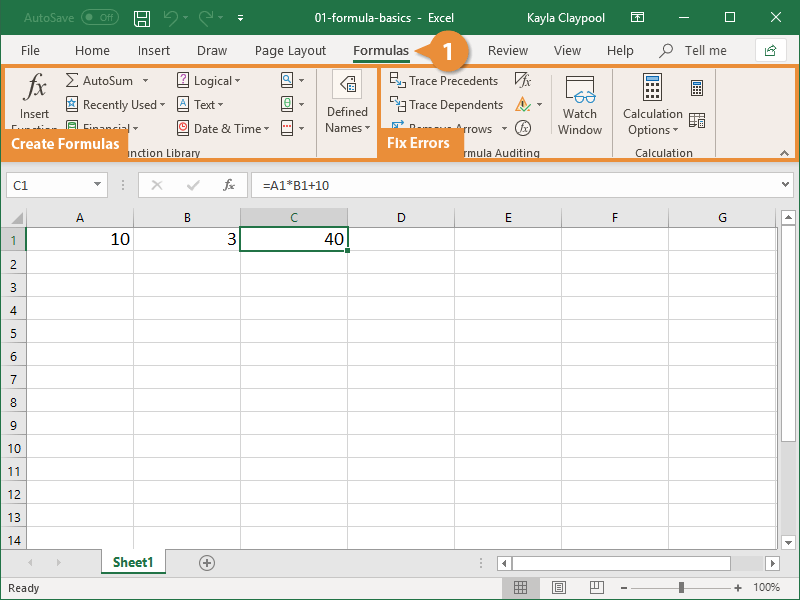Excel Formulas | CustomGuide# Excel Formulas

How to Create Basic Formulas in Excel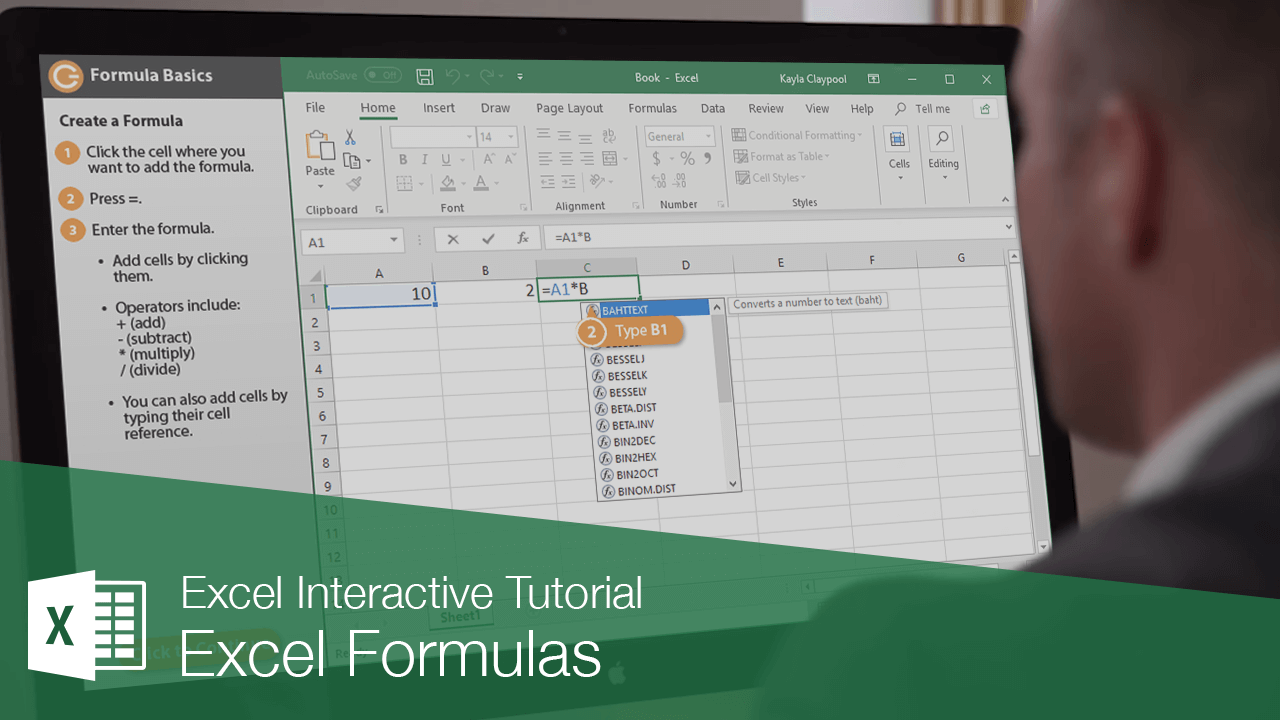Formulas contain information to perform a numerical calculation such as adding, subtracting, or multiplying. All formulas must start with an equal sign (=), followed by:

• Values or cell references joined by an operator. Example: =5+3 or =A1+A2.
• A function name followed by parentheses containing function arguments. Functions are the most common way to enter formulas in Excel. Example: =SUM(A1:A2).

### Create a Formula

Here's how to create a formula in Excel.

1. Click the cell where you want to add the formula.
2. Press =.

Now you must specify what to calculate.

3. Enter the formula.

• Values, like the number 5.
• Cell References, like cell A1. You can add cell references by typing them or clicking the cell you want to reference.
• Operators, or math symbols you want to use in your calculations:
- (subtract)
* (multiply)
/ (divide)
4. Click the Enter button or press Enter when you're finished entering your formula.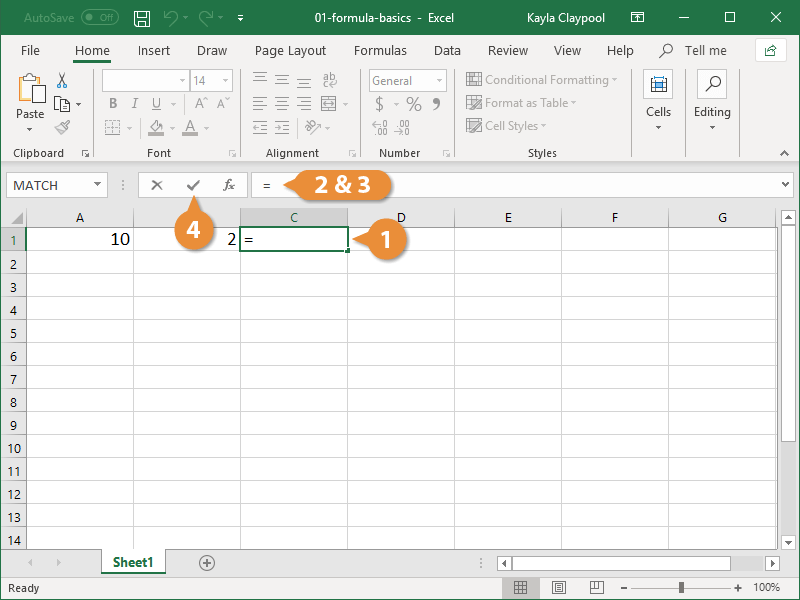If you entered the formula correctly, the results of the formula will appear in the cell.

Operator Formula Example Description
-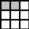=A1-B1 Subtract values.
*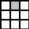=B1*2 Multiply values.
/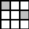=A1/C2 Divide values.
SUM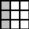=SUM(A1:A3) Totals numbers in a range.
Average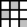=AVERAGE(A2,B1,C3) Averages numbers in a range.

### Automatic Recalculation

If you change the data referenced in a formula, Excel will automatically recalculate the formula to include the updated data.

1. Change the values of the cells that are referenced in a formula.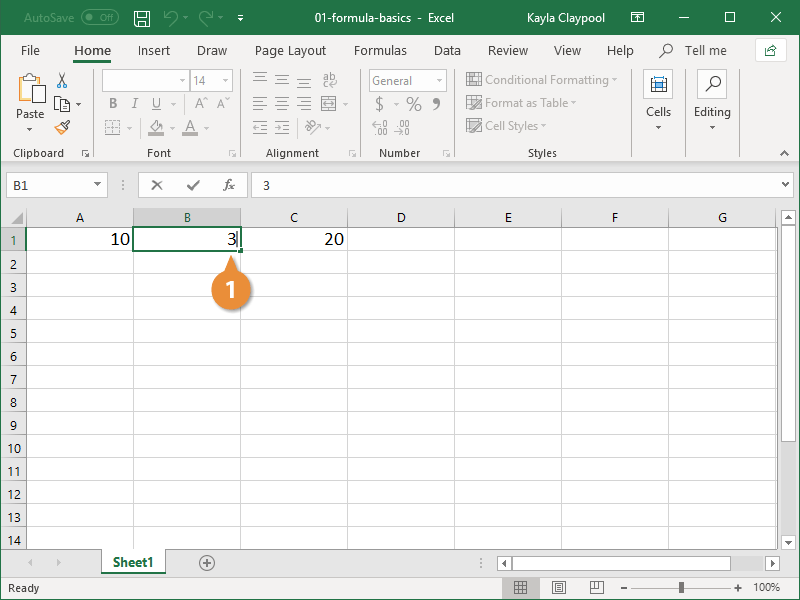Any formulas are automatically recalculated with the changes.

### Edit a Formula

It's easy to make changes to a formula too.

1. Click the formula you want to edit.
2. Click in the Formula bar.
3. Edit the formula.
4. Press Enter.